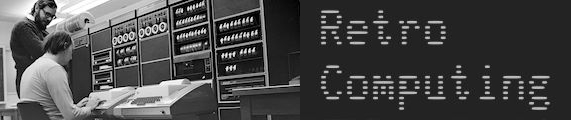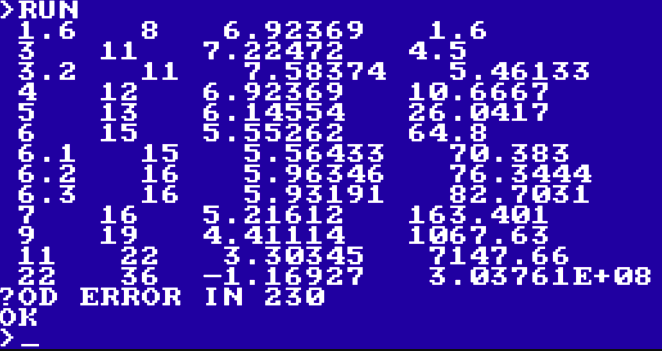# Computing sin(x) in Basic

An interesting little adventure prompted by a post on Huntrods’ Blog:
Z80, Taylor Series for sin(x) and why limits matter

What’s reported here is that accuracy of sin(x) computed with Taylor’s series (the usual power series, I suppose: odd powers of the angle divided by the odd factorials) falls off beyond pi.

That observation goes against my understanding, which is that these power series converge for all arguments. I suspected that what’s happening is that larger arguments cause some of the terms to get quite large, which in any given precision of arithmetic causes some loss of the small parts of those terms. And yet, as alternate terms are mostly cancelling in these cases, the small parts are going to wind up quite important in the final result.

I tested a small program using RISC OS PICO on a Raspberry Pi - helpfully, there are two versions of BBC Basic on this platform, one with 5 byte floats and the other with 8 byte floats. I expected the 8 byte version to do somewhat better for problematic arguments, which would indicate that it’s not the power series that’s unreliable, it’s just the limitations of the usual floating point arithmetic. You need more precision for the calculations than you wanted in the results, if you’re going to evaluation on large arguments.

(The usual practice is to reduce the arguments to a nice small number, by subtracting multiplies of 2pi, then multiples of pi/2 with adjustments to the argument, and then perhaps halving the argument repeatedly and making use of the half-angle formula to reconstruct the final result. So, never evaluate the power series on large arguments!)

One thing I wanted to do was see how large the largest term would be.

Here’s my code. You’ll see I’ve used meaningful variable names throughout, and lots of helpful comments:

``````  100 REM EXPLORE POWER SERIES FOR SIN
110 REM TEST CASES SIN OF 7, 11, AND 22
120 REM TRY 5 AND 8 BYTE FLOATS
130 REM USING 5 BYTE FLOATS:
140 REM SIN(6.2831853), 1 SIG FIG
150 REM SIN(7),   7 SIG FIG
160 REM SIN(11),  4 SIG FIG
170 REM SIN(22),  0 SIG FIG
180 REM USING 8 BYTE FLOATS:
190 REM SIN(6.2831853), 6 SIG FIG
200 REM SIN(7),  14 SIG FIG
210 REM SIN(11), 12 SIG FIG
220 REM SIN(22),  6 SIG FIG
230 A=6.2831853
240 PRINT "CALCULATING SIN OF ";A
250 T=A
260 M=ABS(T)
270 A=A*A
280 S=T
290 F=-1
300 N=2
310 REPEAT
320 T=T*A/N/(N+1)
330 N=N+2
340 PRINT T
350 IF ABS(T)>M THEN M=ABS(T)
360 P=S
370 S=S+F*T
380 F=-F
390 UNTIL S=P
400 PRINT "SIN IS ";S
410 PRINT "MAX TERM WAS ";M
``````

It’s interesting that the blogger found that single vs double precision wasn’t making a difference - that’s something I don’t yet understand.

2 Likes

There are some interesting convergence graphs in “Approximations for Digital Computers” by Cecil Hastings Jr (Princeton, New Jersey, Princton University Press, 1955 – various copies can be found by a Web search.)

Small anecdote, regarding the number of terms used in the series: The original Spacewar code for the PDP-1 uses a four terms series, while a later version for a much faster PDP with the same word length, uses just a three terms series. Apparently, it was found that more algorithmic precision wasn’t worth it, provided you reduced the argument to a small value. (Since sin x approaches x for small numbers and terms like x^9/9! approach zero for small numbers in low precision, few algorithms tend to extend beyond four terms.)

1 Like

I’ll have to have a look for that. Edit: at the IA here.

Of course, I’ve just realised, if you knew the range of input values, you could fix the count of how many terms to sum. And so, if you picked up a recipe which sums a certain number of terms, and applied it on an extended range, you’d see poor accuracy.

Thanks for the detail on Spacewar! Indeed, the precision of trig for gaming needn’t be great. (The original Elite uses a 32-entry lookup table with just one byte of precision.)

I revised my program a bit. I did realise a couple of things

• the program explores how many terms are needed to converge and then looks at accuracy, which doesn’t match the likely case, of fixing the number of terms
• I’m summing lots of terms from the power series, whereas the usual approach is not to truncate the power series but to adjust the coefficients to improve the accuracy over some chosen range

Anyhow, I found a KC85 emulator with four-byte floats, like the usual single precision, and that extends the experiment. (It supports drag-and-drop to load a program, which is very handy.)As you see(!) it takes 8 terms to compute an accurate sine up to pi/2, but 11 terms to compute up to pi, and 16 terms to compute up to 2pi. Even then, the accuracy drops from about 7 digits to less than 6 digits of precision. You can see that the largest term when computing sin(6) is over 64, so that will knock 6 bits off the accuracy of the fractional part.

Here’s the guts of the program as revised:

``````  100 READ A
110 GOSUB 130
120 GOTO 100
130 T=A
140 M=ABS(T)
150 S=T
160 N=2
170 T=-T*A*A/N/(N+1)
180 IF ABS(T)>M THEN M=ABS(T)
190 N=N+2
200 P=S
210 S=S+T
220 IF S<>P GOTO 170
230 D=0
240 IF S=SIN(A) GOTO 260
250 D=-LN(ABS(S-SIN(A)))/LN(10)
260 PRINT A;" ";   : REM ANGLE
270 PRINT N/2;" "; : REM NUMBER OF TERMS
280 PRINT D;" ";   : REM SIGNIFICANT DIGITS
290 PRINT M;" ";   : REM LARGEST TERM
300 PRINT
310 RETURN
320 DATA 1.6,3,3.2,4,5,6
330 DATA 6.1,6.2,6.3,7,9,11,22``````
1 Like

Just for completeness, here’s a table of results:

angle terms sig.fig. terms sig.fig. terms sig.fig. largest
4 byte floats 5 byte floats 8 byte floats
1.6 8 6.9 9 9.6 12 exact 1.6
3 11 7.2 12 9.5 15 16.3 4.5
3.2 11 7.6 13 9.7 16 15.1 5.5
4 12 6.9 13 9.6 17 15.2 10.7
5 13 6.1 15 8.7 19 14.7 26
6 15 5.6 17 8.5 21 16 64.8
6.1 15 5.6 17 8.0 21 14.9 70.4
6.2 16 6 18 8.1 22 14.3 76.3
6.3 16 5.9 18 8.5 22 13.9 82.7
7 16 5.2 18 7.7 22 14.7 163.4
9 19 4.4 21 6.8 26 12.9 1067
11 22 3.3 24 5.5 29 12.7 7147
22 36 -1 41 1.5 47 7.3 3.00E+08
1 Like

Just two notes:

• The KC85 BASIC implementation should be the same as the one for the TRS 80 Model 100.

• My observation on higher order terms was based on 18-bit fixed point math. (Obviously, it doesn’t make sense for floating point arithmetic.)

It may be interesting to think of the Taylor series in terms of graphs: It’s a well known fact that sin x approaches x for very small numbers. What could we do to extend the range, where the straight line graph closely matches the function? Make it a (shallow) curve. This is better, but our graph soon starts to bend away from the actual function graph. Let’s add a correcting term. This is much better, but now we overshoot towards the other side. Let’s add another term, and another term. With each term added, we extend the range of the close fit a bit, by bending the graph slightly towards the opposing curvature. Ideally, repeat until adding another term doesn’t contribute to the result in a given precision.
Notably, any of the higher order terms will approach zero for very small numbers, for all practical means, thus providing correction mostly for bigger values and extending the fitting range.
This may be absolute nonsense in mathematical terms, but this is how I comprehend it.(First, sorry for coming up with Spacewar yet again, but this is where I did my research on binary low level computations.)

Regarding precision in polynomials and practical consequences, see this graph of the gravity calculations of Spacewar 3.1, using fixed point math, and the revised version of Spacewar 4, using on-the-fly conversions to floating point and back again:

Mind the devil’s staircase graph of version 3.1 for small values at the left side, which is owed to the limited precision of fixed point math.
(The formula is f(x,y) = ( √((x >> 3)^2 + (y >> 3)^2) × ((x >> 3)^2 + (y >> 3)^2) ) >> 3, here with x = y, meaning, with all the squares and shifts applied, the remaining precision is extremely limited, especially for small numbers, where it’s about 3 bits of resolution only with fixed-point 18-bit values.)

I think, this is a nice illustration of problems arising from limited precision and how results typically deviate from the ideal function graph. In the case of sine, we may expect a mirrored image, where deviation increases with the size of input values.

(The graph is from https://www.masswerk.at/spacewar/inside/insidespacewar-pt6-gravity.html, where there’s also an extensive excursus on the particular implementation of binary arithmetic, including integer square roots and sine/cosine.)

1 Like

Thanks for this Ed. As the person who wrote the blog, it was frustrating that there was so little information available regarding ‘actual’ results. I looked and could not find any info as to why the results > 2PI were so bad.

Your post answers that question. I was using 5 terms in the series, so even with the ‘best’ precision on a real Z80 computer, -2PI to 2PI was the range where values were reasonable.

I will try some more playing with more terms (it’s just a constant in the program) and see what I get.

Meanwhile I continue to play with PL/I on the Z80. It’s amazing what decent compilers I can get for CP/M 2.2 with minimal effort compared to the digging I’ve had to do to get older compilers for ‘modern’ machines. As it is I can’t seem to find a working PL/I compiler (there is one, but all the zips are corrupted).

2 Likes